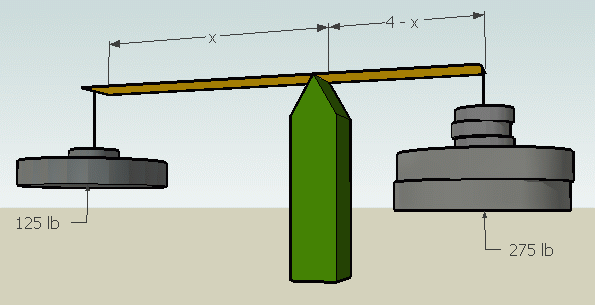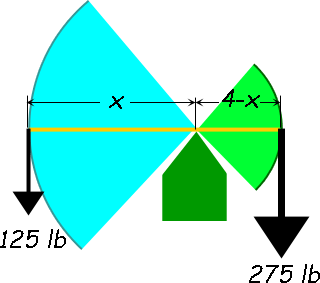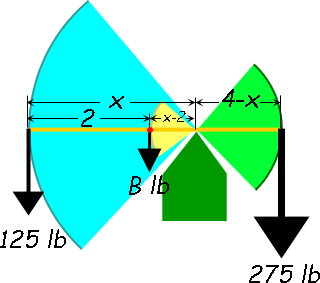SEARCH HOMEMath Central Quandaries & QueriesQuestion from Eric: If I have a 4" bar that has no weight value with a 275lb. weight on one side and a 125lb. weight on the other side what would be the folcrum point? Please keep the answer very simple. Also is there a fixed equation to figure folcrum points? Is there an equation for if the 4" bar had a weight value?Hi Eric.

Let's say x is the number of inches to place the fulcrum from the end that holds the 125 lb.This question concerns torque. The net torque must be zero for the bar to remain steady. So start by drawing a diagram of the forces involved.

Weightless bar:You can see that the lever arms are different lengths in my diagram. Since torque equals force times the lever arm, we have

 Net counter-clockwise torque = Net clockwise torque 125(x) = 275(4-x)

and must simply solve for x.

Bar with weight:

If the bar is not weightless, you need to consider the extra torque applied by the bar acting at its own center of mass (for a uniform bar, this is the midpoint of the bar).

You would need to know the weight of the bar of course. Let's say this is B. Then to balance the torques we have:

 Net counter-clockwise torque = Net clockwise torque 125(x) + B(x-2) = 275(4-x)

And again, we'd solve for x.

Hope this helps,
Stephen La Rocque.Math Central is supported by the University of Regina and The Pacific Institute for the Mathematical Sciences.